﻿ 森林在递归算法分析中的应用 Application of Forest in the Analysis of Recursive Algorithm

Computer Science and Application
Vol. 09  No. 07 ( 2019 ), Article ID: 31408 , 8 pages
10.12677/CSA.2019.97157

Application of Forest in the Analysis of Recursive Algorithm

Yuekun Liu, Sunying Zhuan, Beining Xu, Bihe Shen

School of Information and Control Engineering, Qingdao University of Technology, Qingdao Shandong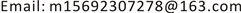Received: Jul. 4th, 2019; accepted: Jul. 18th, 2019; published: Jul. 25th, 2019ABSTRACT

This paper presents a method of recursive algorithm analysis by forest. This method maps recursive logic to forest. Tree is used as processing unit. The recursive structure of program is analyzed and tree nodes are defined. Tree is established by searching and calling state table. The tree is not limited to binary tree. According to practical problems, it can be composed of single branch tree, binary tree, multi-branch tree or even multi-tree. According to the implementation of the program, many trees need to jump between trees. According to the comparison between the tree and the invocation state table, the analysis is comprehensive, and the analysis structure is clear, the results are accurate, and the application is extensive, which effectively solves the difficulties in the analysis of the results of the recursive algorithm.

Keywords:Forest, Logistic Map, State Table, Recursive Algorithm, Search, BacktrackCopyright © 2019 by author(s) and Hans Publishers Inc.1. 引言

2. 树在递归分析中的应用

(1)void Recursive (int m, int n)

{

(2) if (m==0)

(3) return ;

(4) if (n==0)

{

(5) a= Recursive (m-1,1);

(6) return a;

}

(7)else

{

(8) b= Recursive (m-1,n);

(9) printf(“%d %d”,m,n);

(10) c= Recursive (m,n-1);

}

}

2.1. 分析程序结构

2.2. 建立树型图

2.3. 建立状态调用表

3. 实例分析

3.1. 汉诺塔问题

void hanoi (int n,char a,char b,char c)

{

if(n==1)

{

printf(“%c-->%c”, a,c);//1

}

else

{

hanoi(n-1,a,c,b );

printf(“%c-->%c”, a,c);//2

hanoi(n-1,b,a,c);

}

}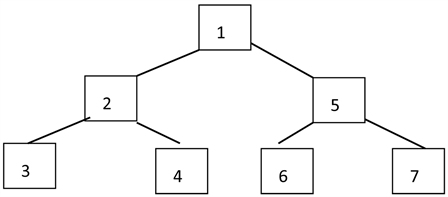Figure 1. The tree structure of the Tower of Hanoi

Table 1. The Tower of Hanoi call status table on execution procedure

3.2. 2018 noip普及组试题三(3)

int findans(int n,int m)

{

if(n==0) return m;

if(m==0) return n%3;

return findans(n-1,m)-findans(n,m-1)+findans(n-1,m-1);

}

f1= findans(n-1,m)

f2= findans(n,m-1)

f3= findans(n-1,m-1)

return f1-f2+f3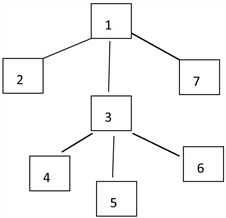Figure 2. The tree structure of 2018 noip popularization groupTable 2. The call status table of 2018 noip popularization group

3.3. 阿克曼函数

n+1m=0,n>0

A(m,n)=A(m-1,1) n=0,m>0

A(m-1,A(m,n-1)) n>0,m>0

int Ackerman(int m, int n)

{

if (m==0)

return n + 1;

if (n==0)

return Ackerman(m - 1, 1);

else

return Ackerman(m - 1,Ackerman(m, n - 1));

}

a= Ackerman(m, n - 1);

b= Ackerman(m - 1,a);

return b;

t1调用树结构如图3所示，执行过程调用状态如表3所示，跳转后的分支树t2的调用树结构如图4所示，执行过程调用状态如表4所示。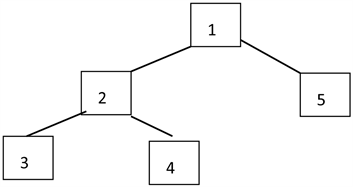Figure 3. The tree structure of t1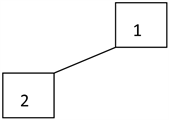Figure 4. The tree structure of t2

4. 结论

Application of Forest in the Analysis of Recursive Algorithm[J]. 计算机科学与应用, 2019, 09(07): 1398-1405. https://doi.org/10.12677/CSA.2019.97157

1. 1. Prata, S. C Primer Plus [M]. 第6版. 姜佑, 译. 北京: 人民邮电出版社, 2016: 256-282.

2. 2. 晏素芹. 递归算法的教学方法探讨——以C程序设计为例[J]. 福建电脑, 2018, 34(8): 170-171.

3. 3. 方娇莉, 潘晟旻, 刘明. 程序设计微课教学设计方法研究——以递归为例[J]. 计算机教育, 2016(5): 116-120.

4. 4. 吴晓晨. 递归程序设计教学方法的研究[J]. 天津科技, 2017, 44(1): 69-71.

5. 5. 陈瑞环, 杨庆红, 姚兴. 使用递推解决递归问题的研究与应用[J]. 计算机应用与软件, 2011, 28(3): 186-187.

6. 6. 周法国, 韩智, 高天. 递归算法设计思想与策略分析[J]. 软件导刊, 2017, 16(10): 35-38.

7. 7. 张建波. 一种将递归过程转换为非递归过程的方法研究[J]. 计算机教育, 2017(8): 139-142.

8. 8. 黎远松. 基于树的递归算法分析技术[J]. 四川理工学院学报(自然科学版), 2012, 25(4): 50-51.

9. 9. 张俊. 基于递归树的递归调用分析[J]. 实验室研究与探索, 2010, 29(3): 83-87.

10. 10. 严蔚敏, 吴伟民. 数据结构(C语言版) [M]. 北京: 清华大学出版社, 2009: 118-155.